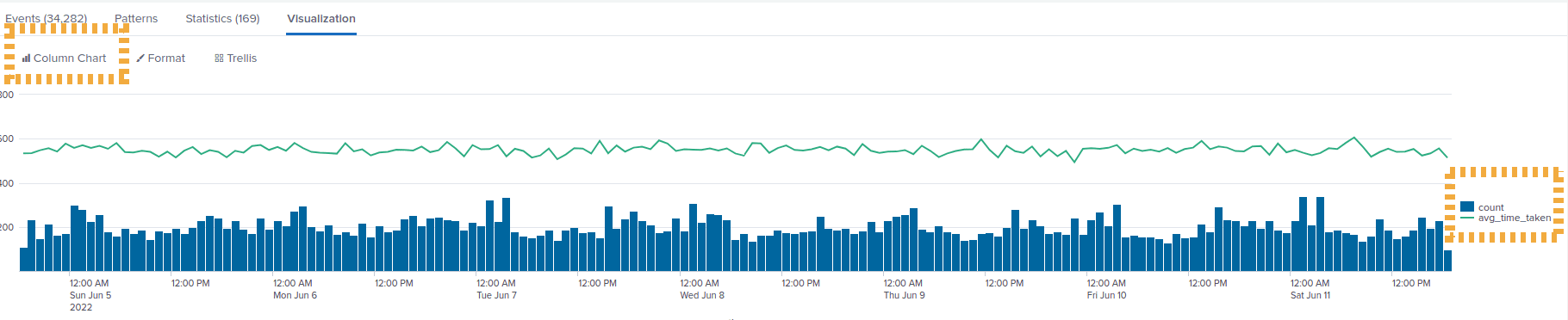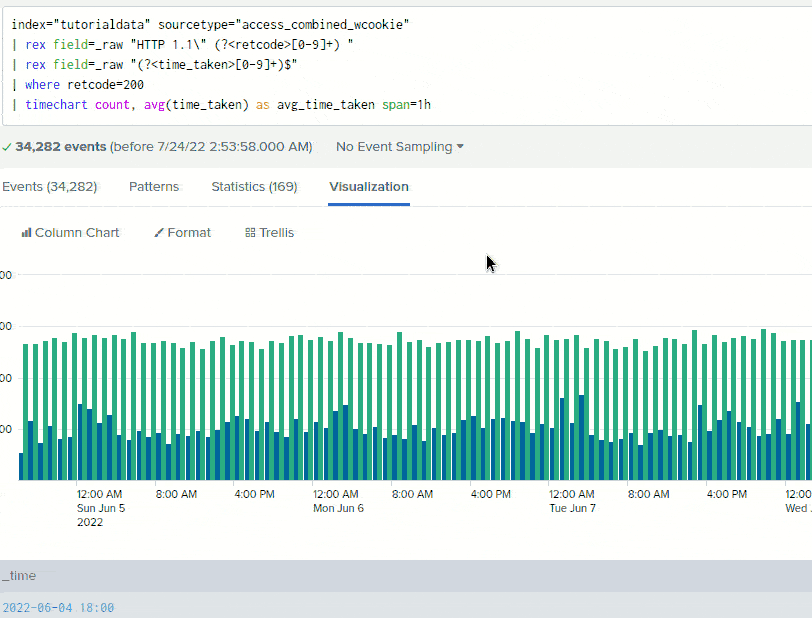Splunk Examples: Timecharts

# Splunk Examples: Timecharts

Last updated:

Splunk version used: 8.2.6.

## Custom period

To set a custom step size in timecharts, use span=<period> after timechart:

Example: group by 5-minute buckets, count rows

source=logs "some-search-criteria"
| timechart span=5m count


TODO redo using tutorial data, add screenshots

## Group by value, count by period

Here you can extract a value using rex first and then apply count by:

Example: Count how many occurrences of each type of purchase by period

source=logs "new-purchase-made"
| rex "purchase-type: (?<type>\w+) "
| timechart count by type


TODO redo using tutorial data, add screenshots

## Bars and lines in the same chart

Examples use the tutorial data from Splunk

This is useful if you want to plot something like the amount of requests (as bars) and the average response time (line) on the same chart.

You want to use Chart Overlays for that.

Using the tutorialdata, create a query with a timechart

index="tutorialdata" sourcetype="access_combined_wcookie"
| rex field=_raw "HTTP 1.1\" (?<retcode>[0-9]+) "
| rex field=_raw "(?<time_taken>[0-9]+)\$"
| where retcode=200
| timechart count, avg(time_taken) as avg_time_taken span=1h


Select Column Chart as the chart type (for the count attribute) and then add the other attribute avg_time_taken as an Overlay:A splunk timechart with bars and lines together in the same plotConfiguring the overlay option on
Splunk visualization